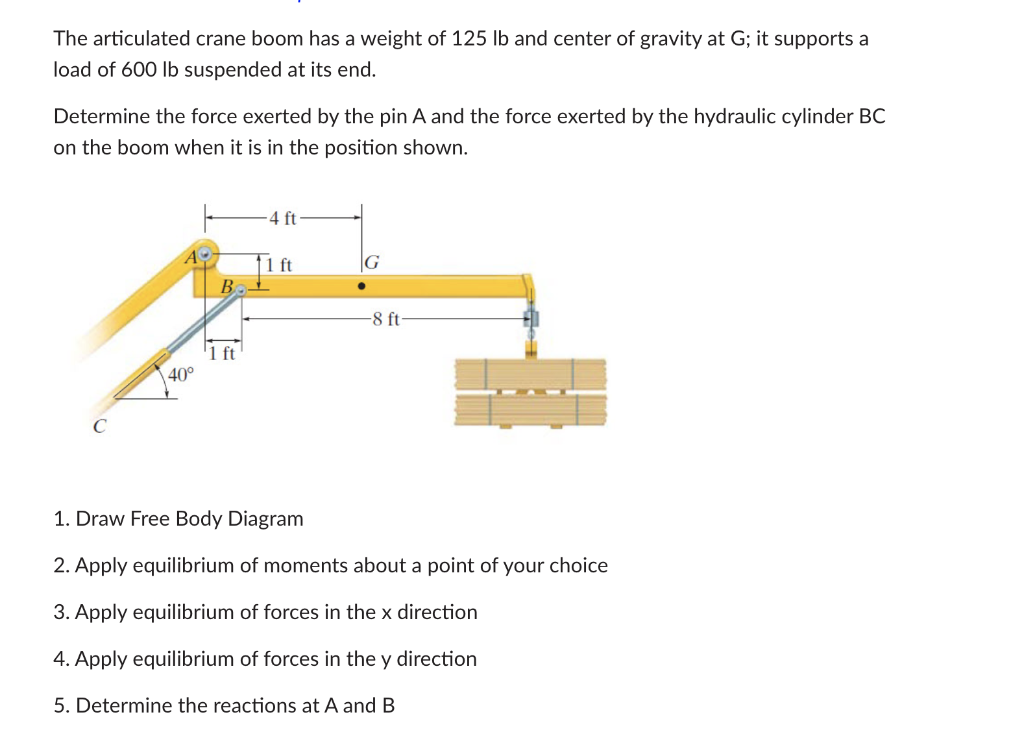# Question The articulated crane boom has a weight of 125 lb and center of gravity at G; it supports a load of 600 lb suspended at its end. Determine the force exerted by the pin A and the force exerted by the hydraulic cylinder BC on the boom when it is in the position shown. 4 ft 11 ft G B -8 ft '1 ft 40° 1. Draw Free Body Diagram 2. Apply equilibrium of moments about a point of your choice 3. Apply equilibrium of forces in the x direction 4. Apply equilibrium of forces in the y direction 5. Determine the reactions at A and B5XIIPJ The Asker · Mechanical EngineeringTranscribed Image Text: The articulated crane boom has a weight of 125 lb and center of gravity at G; it supports a load of 600 lb suspended at its end. Determine the force exerted by the pin A and the force exerted by the hydraulic cylinder BC on the boom when it is in the position shown. 4 ft 11 ft G B -8 ft '1 ft 40° 1. Draw Free Body Diagram 2. Apply equilibrium of moments about a point of your choice 3. Apply equilibrium of forces in the x direction 4. Apply equilibrium of forces in the y direction 5. Determine the reactions at A and B
More
Transcribed Image Text: The articulated crane boom has a weight of 125 lb and center of gravity at G; it supports a load of 600 lb suspended at its end. Determine the force exerted by the pin A and the force exerted by the hydraulic cylinder BC on the boom when it is in the position shown. 4 ft 11 ft G B -8 ft '1 ft 40° 1. Draw Free Body Diagram 2. Apply equilibrium of moments about a point of your choice 3. Apply equilibrium of forces in the x direction 4. Apply equilibrium of forces in the y direction 5. Determine the reactions at A and B